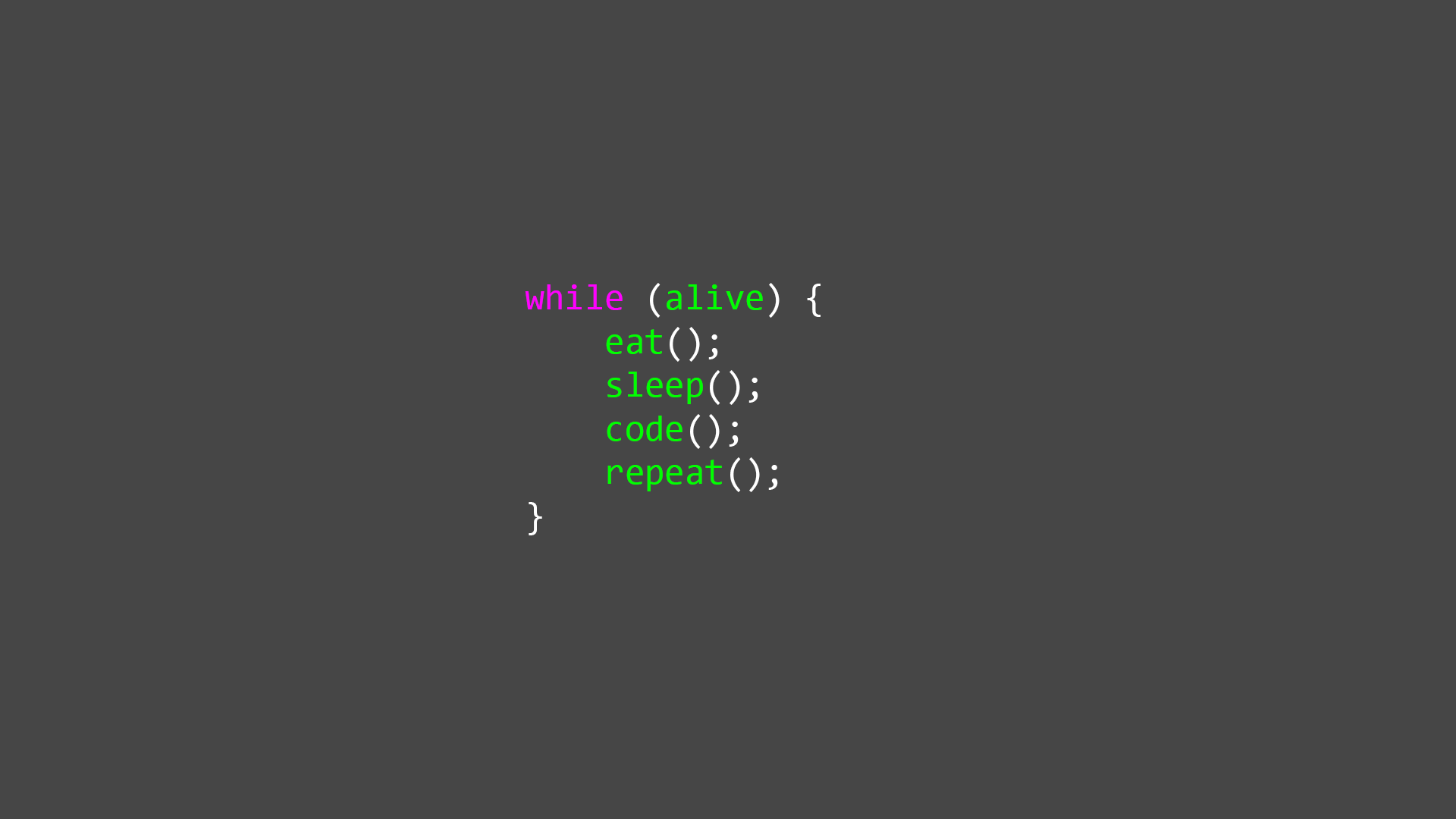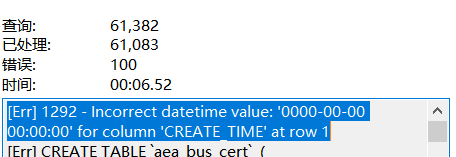C语言中指针及其应用高级篇（用指针实现数组的扩增）• 阅读 1104

# 一、一级指针与一维数组

``````#define _CRT_SECURE_NO_WARNINGS
#include<stdio.h>
#include<stdlib.h>
#include<string.h>
#include<malloc.h>
int main() {

//把指针充当变量的方式有两种
//1. 通过变量的地址初始化
int num = 1;
int *pInt = &num;
*pInt = 101;    //这里的*pInt等效于num
printf("*pInt=%d\tnum=%d\n", *pInt, num);

//2.1. 通过使用malloc初始化内存，让指针变成普通变量
int *p = (int *)malloc(sizeof(int));
if (p == NULL) {
return 0;
}
*p = 101;
printf("*p=%d\n", *p);
free(p);
p = NULL;

//2.2 可以把指针变成数组，一级指针则成为一维数组
int *array = (int *)malloc(sizeof(int ) * 3);
if (array == NULL) {
return 0;
}
for (int i = 0; i < 3; i++) {
scanf_s("%d", array + i);    //把从键盘输入的字符存储到数组中
//array[i] = i;   自动添加数字元素到数组中
printf("arr[%d]=%d,arr[%d]=%d\n", i, array[i], i, *(array + i));
}
free(array);
array = NULL;

//3. 指针作为字符数组
char *pChar = (char *)malloc(sizeof(char) * 27);
for (int i = 0; i < 26; i++)
{
pChar[i] = 'A' + i;
printf("%c\t", pChar[i]);
}
printf("\n");

//3.1 字符数组，字符串的区别
pChar = '\0';
printf("%s\n", pChar);
printf("\n");
free(pChar);
pChar = NULL;

//3.2 关于字符数组赋值的问题,C语言中没有string类型
char *pStr = (char *)malloc(sizeof(char) * 10);   //pStr;
/*
字符串的赋值只能通过两种方式
1. 字符串采用字符的方式去处理
2. 通过字符串拷贝函数去赋值
*/
strcpy(pStr, "Loveyou");
printf("%s\n", pStr);
free(pStr);
pStr = NULL;

//3.3 申请的数组长度可以为一个变量
printf("你想输入多少个学生信息:");
int count = 0;
scanf_s("%d", &count);
float *pScore = (float *)malloc(sizeof(float)*count);
printf("请输入%d个学生成绩\n", count);
for (int i = 0; i < count; i++)
{
scanf("%f", pScore + i);
}
for (int i = 0; i < count; i++)
{
printf("%f\t", pScore[i]);
}
printf("\n");
free(pScore);
pScore = NULL;
system("pause");
return 0;
}``````

#### 2.1使用malloc函数使指针变量变成普通变量

``int *p = (int *)malloc(sizeof(int));``

``````  free（指针变量名字）;
指针变量名=NULL;``````

#### 2.2使用 malloc申请一段内存

``int *array = (int *)malloc(sizeof(int ) * 3);``

#### 2.3 使用malloc申请字符类型的内存

``char *pChar = (char *)malloc(sizeof(char) * 27);``

``````printf("%c\t", pChar[i]);
pChar = '\0';``````

# 二、二级指针与二维数组

``````#define _CRT_SECURE_NO_WARNINGS
#include<stdio.h>
#include<stdlib.h>
#include<string.h>

//1.2  通过返回值申请内存，这是一个申请数组的函数
int *Mallo_Array(int arrLength) {
int *array = (int *)malloc(sizeof(int *)*arrLength);
return array;    //返回数组首地址
}
//1.3  通过参数申请内存
void  malloc_Array1D(int **array, int arrLen) {
//通过一级指针修改普通变量的值，因此改变普通变量时候传递一级指针
//通过二级指针修改一级指针的指针变量，因此改变指针指向的时候要传递二级指针
*array = (int *)malloc(sizeof(int  *)*arrLen);
}

//1.4  字符串当做函数参数，申请内存
//通过外部变量来初始化字符串，如申请一个内存存储字符串，可能传一个常量，而常量是不能修改的，因此要申请一段内存来保存常量
//这里不用malloc而是用 strlen，因为fileName只是一个指针，用malloc申请到的永远是4个字节
void malloc_String(const char *fileName) {
char *pStr = (char *)malloc(strlen(fileName) + 1);        //计算长度，此处字符串数组长度要+1
strcpy(pStr,fileName);        //使用strcpy来赋值，把fileName拷贝到pStr中就可以对fileName进行修改操作
printf("%s", pStr);
}

int main() {
//1.1 二级指针和二维数组
int *pInt = (int *)malloc(sizeof(int) * 3);        //意味着每一个SecPointer[i]都是一个一级指针
int **SecPointer = (int **)malloc(sizeof(int *) * 9);    //开辟九个长度的存放一级指针变量的数组
for (int i = 0; i < 9; i++) {
SecPointer[i] = (int *)malloc(sizeof(int) * 9);        //为九个指针申请内存使其成为二维数组
}
SecPointer = 101;
printf("%d\n", SecPointer);
free(SecPointer);
SecPointer = NULL;

//1.2  调用函数申请一个容量为3的一维数组
int *array = Mallo_Array(3);
for (int i = 0; i < 3; i++) {
array[i] = i;
printf("array[%d]=%d,array[%d]=%d\n", i, *(array+i),i,array[i]);
}
free(array);
array = NULL;

//1.4
malloc_String("LoveYou");
printf("\n");
system("pause");
return 0;
}``````

# 三、malloc，realloc，calloc

``````#include<stdio.h>
#include<stdlib.h>

//1.1  calloc函数在申请内存后会默认初始化
//1.2  realloc函数可在内存不足的情况下重新申请内存，且具有复制功能，即会把原来内存里的元素再复制到新申请的内存中

int main() {
//1.1
int *pCalloc = (int *)calloc(3, sizeof(int));
int *pMalloc = (int *)malloc(sizeof(int) * 3);
for (int i = 0; i < 3; i++) {
printf("Calloc[%d]=%d\n", i, pCalloc[i]);
}
printf("\n");
free(pCalloc);
pCalloc = NULL;
for (int j = 0; j < 3; j++) {
printf("Malloc[%d]=%d\n", j, pMalloc[j]);
}
printf("\n");
free(pMalloc);
pMalloc = NULL;

//1.2
int *pMall = (int *)malloc(sizeof(int) * 3);
for (int x = 0; x < 3; x++) {
pMall[x] = x;
}
realloc(pMall, sizeof(int) * 6);
for (int y = 3; y < 6; y++) {
pMall[y] = y;
}
for (int z = 0; z < 6; z++) {
printf("%d", pMall[z]);
}
system("pause");
return 0;
}``````

``````int *pCalloc = (int *)calloc(3, sizeof(int));
int *pMalloc = (int *)malloc(sizeof(int) * 3);``````
``````int *pMall = (int *)malloc(sizeof(int) * 3);
realloc(pMall, sizeof(int) * 6);``````

## 球球了，给点个赞吧 !（>-<）!

**Jacquelyn38
1年前
2020年前端实用代码段，为你的工作保驾护航blmius
1年前
MySQL:[Err] 1292 - Incorrect datetime value: ‘0000-00-00 00:00:00‘ for column ‘CREATE_TIME‘ at row 1Stella981
11个月前
PhoneGap设置IconStella981
11个月前
KVM调整cpu和内存Wesley13
11个月前
MySQL总结(十一)子查询
![](https://oscimg.oschina.net/oscnet/up-a344f41e81d3568e3310b5da00c57ced8ea.png) 子查询 === * * * 1\. 什么是子查询 ---------- -- 需求：查询开发部中有哪些员工 select * from emp; -- 通Easter79
11个月前Wesley13
11个月前
MySQL查询按照指定规则排序
1.按照指定(单个)字段排序 select * from table_name order id desc; 2.按照指定(多个)字段排序 select * from table_name order id desc,status desc; 3.按照指定字段和规则排序 selecStella981
11个月前
Angular material mat
Icon Icon Name mat-icon code _add\_comment_ add comment icon <mat-icon> add\_comment</mat-icon> _attach\_file_ attach file icon <mat-icon> attach\_file</mat-icon> _attach\Wesley13
11个月前
MySQL部分从库上面因为大量的临时表tmp_table造成慢查询
#### 背景描述 # Time: 2019-01-24T00:08:14.705724+08:00 # User@Host: **[**] @ [**] Id: ** # Schema: sentrymeta Last_errno: 0 Killed: 0 # Query_time: 0.315758 Lock_helloworld_34035044
2个月前

​在我们用皕杰报表工具设计填报报表时，如何在新增行里自动增加id呢？能新增整数排序id吗？目前可以在新增行里自动增加id，但只能用uuid函数增加UUID编码，不能新增整数排序id。 uuid函数说明：获取一个UUID，可以在填报表中用来创建数据ID语法：uuid() 或 uuid(sep)参数说明：sep 布尔值，生成的uuid中是否包含分隔符''，缺省为Lv1

25

3

9